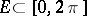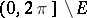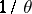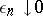# Uniqueness set

(diff) ← Older revision | Latest revision (diff) | Newer revision → (diff)-set
A setsuch that a trigonometric series that converges to zero at each point ofis the zero series. A set that is not a-set is a called a set of non-uniqueness, or an-set. These concepts are related to the problem of the uniqueness of the representation of a function by a trigonometric series converging to it everywhere, except perhaps on a given set. G. Cantor (1872) showed that a finite set (including the empty set) is a set of uniqueness, and the extension of this result to infinite sets led him to the creation of set theory.
Sets of positive Lebesgue measure are always-sets. Any countable set is a-set. There exists perfect sets (cf. Perfect set) of measure zero that are-sets (D.E. Men'shov, 1916), and ones that are-sets (N.K. Bari, 1921); for example, the Cantor set with a constant rational ratiois a-set if and onlyis an integer, that is, whether a set of numbers is a-set or an-set depends on the arithmetical nature of the numbers forming it. However, there exist setsof full measure (so-called-sets) such that any trigonometric series that converges to zero at every point ofand has coefficients that are, where, is the zero series.
The concepts of-sets and-sets can be generalized to Fourier–Stieltjes series.